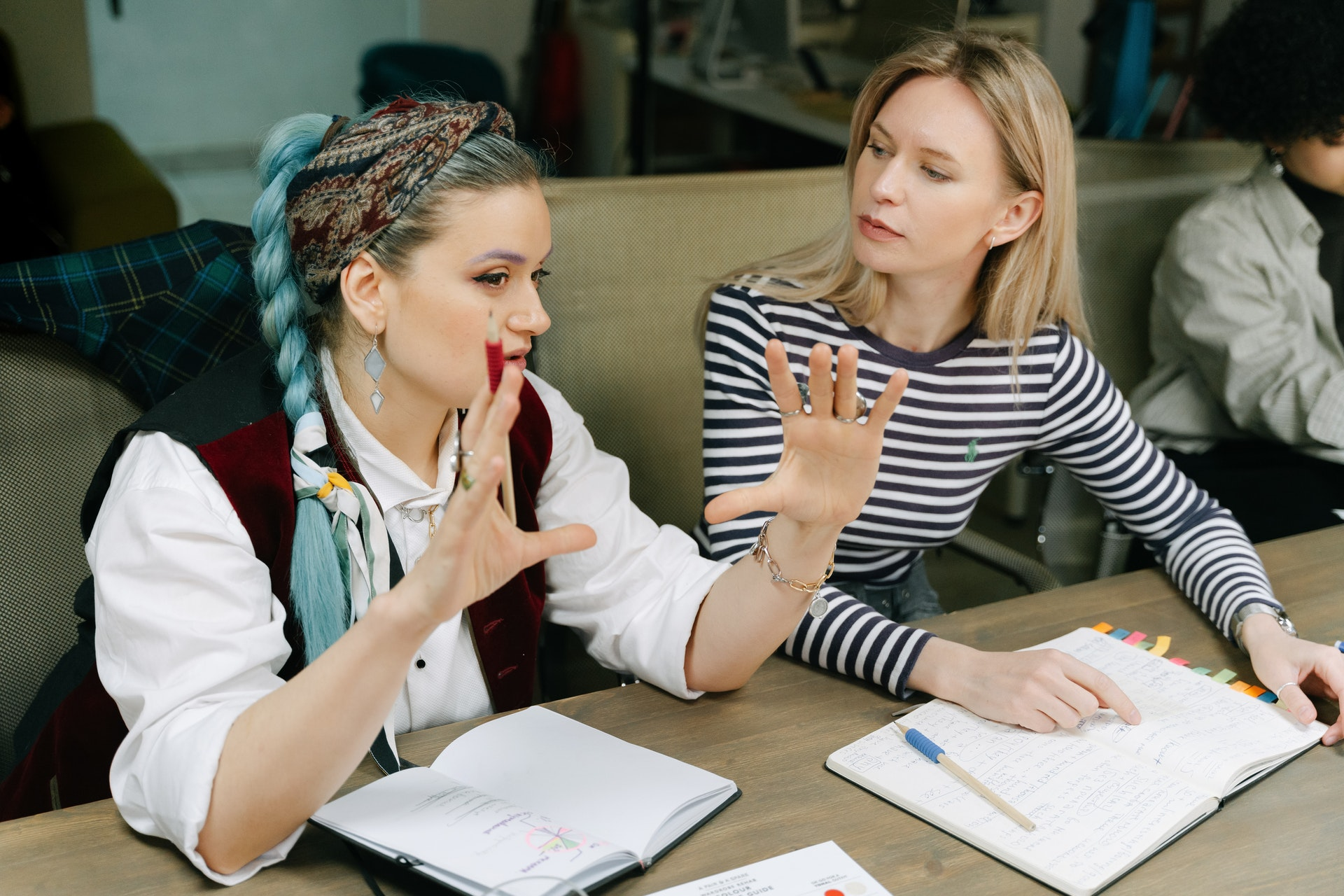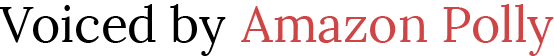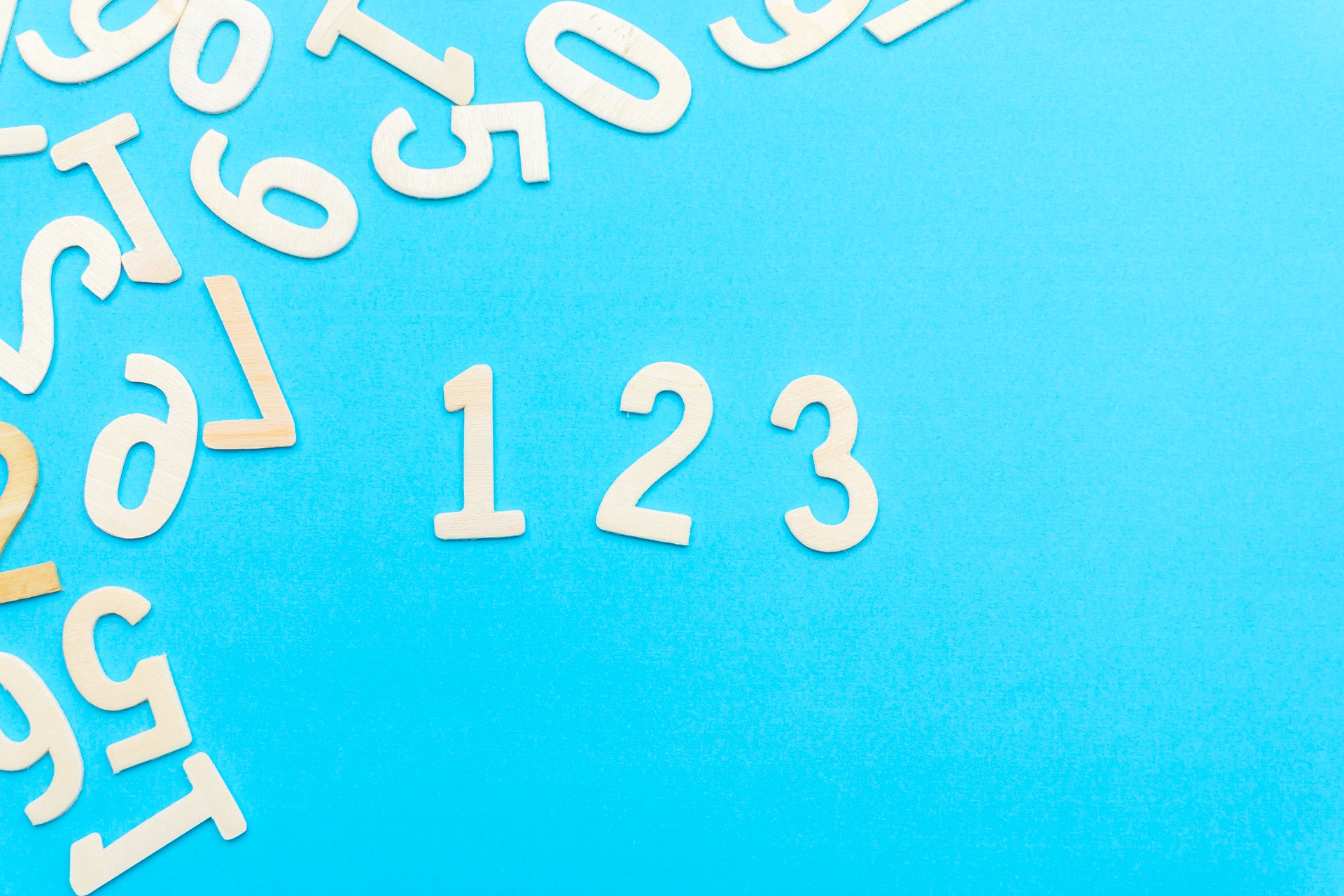# How to Teach Rounding and Estimating

 Would You Rather Listen to the Lesson?Pre-algebra students learn the concept of rounding and estimating numbers. They are thus able to estimate specific numbers with the help of rounding off, and they can also find the sum, difference, and quotient of numbers by using estimation.

And while the process may seem straightforward to many students, others may struggle with rounding off and estimation of numbers. To help out, we bring you a few teaching tips for this topic. Follow these tips and see your students rounding and estimating effortlessly!

## Tips for Teaching Rounding and Estimating

### What Is Rounding?

You can explain that rounding refers to a process of simplifying numbers so that they’re easier to work with. More specifically, when we’re rounding a long number, we’re making it simpler by expressing it in terms of the nearest unit (such as the nearest ten, hundred, tenth, etc.).### How to Round Off Whole Numbers

After providing a general definition, you can provide an outline of the rules for rounding whole numbers. You can enumerate them in the following way on the whiteboard:

1. Identify the rounding digit of the number and determine its place value. Pre-algebra students should already have a good grasp of place value, but if you notice that some are struggling, you may consider reviewing it with the identified students. You may find our article on place value useful for this purpose.
2. After having identified the rounding digit, look at the digit to its right. If this digit is less than 5, we don’t change the rounding digit. However, if the digit to the right of the rounding digit is 5 or greater than 5, we increase the rounding digit by 1.
3. Finally, we change all digits to the right of the identified rounding digit to zero, while retaining all the digits to the left of the rounding digit.

#### Example 1:

Start with an easier example of a number that doesn’t contain too many digits, for example, show students how to round 141 to the nearest ten. You can underline or highlight the rounding digit with a marker to help children visualize which digit we need to round.

In this case, this is 4, as its place value is 10, i.e. 4 is in the tens place and since we’re trying to round off 141 to the nearest ten, we need to focus on the digit that’s in the tens place. You can now show students how we apply the above-mentioned rules in practice.

After having determined that the rounding digit is 4, we then look at the digit to its right, that is, 1. Remind students that the rule states that if the digit to the right of the rounding digit is less than 5, we don’t change the rounding digit.

Point out that since 1 is less than 5, we won’t change the rounding digit, i.e. 4 will stay the same. Finally, we’ll change all digits to the right of the identified rounding digit to zeros. So by rounding 141 to the nearest ten, we’ll get 140.

#### Example 2:

You can now move on to numbers with more digits, such as 421,281. Write this number on the whiteboard and show students how to round it to the nearest hundred. Again, it can be practical to underline or highlight the rounding digit.

Explain that if we want to round off 141,281 to the nearest hundred, we need to look at the digit in the hundreds place, i.e. the digit whose place value is 100. In this case, this is 2. Then, we need to identify the digit to its right, that is, 8.

Point out that since 8 is bigger than 5, we need to apply the above rule, whereby if the digit to the right of the rounding digit is 5 or greater than 5, we increase the rounding digit by 1. That means that we’ll increase the rounding digit 2 by 1 and transform it into 3.

Just like in the previous example, we’ll change all digits to the right of the identified rounding digit to zeros, and we’ll retain all digits to the left of the rounding digit. Thus, by rounding 141 281 to the nearest hundred, we’ll get 421,300.

### What Is Estimating?

Once students are comfortable with rounding, you can explain estimating. This is also a way in which we make numbers easier to work with when we need to find a number that’s close enough to the right answer, but we don’t need the exact answer.

Estimating is a bit different from rounding in that it represents a border type of approximation. With estimating, we’re not just modifying an existing number, but we’re coming up with a new number, for example, when calculating. You can also provide a few practical examples of why and where we may need estimating.

For instance, ask children to imagine they’re in a store where they’re purchasing several food items, and they want to have a general idea of what they’re going to have to pay in the end. Explain that this is a situation where estimating can make life easier.

### How to Do Estimating

#### Estimating with Rounding

Explain to students that rounding is the most commonly used method for estimating. Point out that to estimate the answer to a certain calculation, we first need to round off each individual number using the rules explained earlier.

##### Example:

For example, show students how we can estimate the answer to 921 – 512. We may not always be able to use a calculator for these kinds of operations, but by using mental math, we can estimate the answer.

First, we need to round off each number in the operation to the nearest hundred, that is, 921 and 512. By rounding off 921 to the nearest hundred, we’ll get 900 and by rounding off 512 to the nearest hundred, we’ll get 500.

Then, we’ll simply do the mental math of subtracting 500 from 900, which we’ll give us 400. Finally, you can check what the exact answer is on a calculator. Point out to students that the exact answer to 921 – 512 is 409, which is close to our estimate of 400!

#### Front End Estimation

Explain to students that we can also use another type of estimation, called front-end estimation, to be able to estimate an answer that’s close enough to the exact one. As the name suggests, with front-end estimation, we round the number in the front.

In other words, instead of using the above rounding method where we round each number based on a certain place value, here we only round whatever numbers (or one number) are in the leftmost place. All other numbers will be zeros.

##### Example:

For example, you can show students how to use front-end estimation and estimate the answer to a sum of two numbers, such as 15,345 + 122. Pint out that the first thing that we need to do is identify the number in the leftmost place, i.e. the number with the biggest place value.

In 15,345, this digit is 1 and its place value is 10,000. This is our rounding digit. Then we identify the digit to its right, that is, 5. Since we already know that if the digit to the right of the rounding digit is 5 or greater than 5, we increase the rounding digit by 1, we’ll round 15,345 to 20,000.

Then we repeat the same procedure with the second number in the addition, that is, 122. The digit in the leftmost place is 1, with a place value of 100. So our rounding digit is 1. The digit to its right is 2, which is less than 5.

Remind students that we know that if the digit to the right of the rounding digit is less than 5, we won’t change the rounding digit. The only thing left to do is transform all other numbers to zeros, which means we’ll round 122 to 100.

Then we’ll try to estimate the answer by using the rounded numbers:

20,000 + 100 = 20,100

So by using front-end estimation, we managed to estimate that the answer to 15,345 + 122 is around 20,100. You can also use a calculator to find the exact answer and compare the estimation with the exact answer.

## Activities to Practice Rounding and Estimating### Pair Work

This activity will help students practice their skills of rounding and estimating numbers. To implement the activity in your classroom, the only thing you’ll need is this free Assignment Worksheet. Print out as many copies as needed based on your class (one copy per student).

Pair students up. Make sure that students are paired in an efficient manner, that is, students with stronger math skills should be paired with students who might be struggling in math. This way, students will have the opportunity to engage in peer teaching.

Explain to students that they have to work on the worksheets and try to solve the math problems they contain to the best of their ability. The worksheets contain diverse rounding and estimating problems, including estimating with rounding and estimating with front-end estimation.

You can give students some 15 minutes to solve the problems. After that, they check the work of the student that they’re paired with and try to help with any mistakes they identify. Homeschooling parents can also use the worksheet as an individual exercise.

### Half-Court Rounding Game

This is an online game that will reinforce students’ ability to round off numbers quickly. To implement this game in your classroom, the only thing you need is a sufficient number of devices (one per student), as well as a decent internet connection.

Arrange students in pairs and provide instructions for the game. Each student plays individually and tries to score as many points as possible by rounding the numbers that they’re given. They have 90 seconds to do this, so they need to think fast.

Explain to students that the game consists of a basketball player trying to shoot. Students are the ones who select the shot difficulty, and are then presented with a number to round. The greater the shot difficulty and the number rounding, the more points they score.

Once the student answers the math question, the basketball player shoots the ball automatically and scores points if the answer was correct. If the student answered incorrectly, the basketball player will miss the shot.

In the end, the two students in a pair compare their scores. The one with the biggest score wins the game. They may also decide to engage in another round if time allows it. If you’re a homeschooling parent, you can use the game for individual practice.

### Estimation Valley Golf Game

This is a fun online game that challenges students to think quickly to estimate the answer to a given addition, subtraction, or multiplication problem, depending on the level they choose. Make sure there are suitable devices for every student and launch the game!

Divide students into groups of 3 and explain the rules of the game. The members of one group play individually and compete against each other. They try to quickly solve the math problems in the game, such as providing an estimate to 5267 – 2865.

Explain to students that they need to select a golf player (their character for the game) and a course. The course represents the difficulty of the problems that they’ll be asked to solve, so make sure that each student in a group selects the same course.

For example, by choosing the junior course, they get addition and subtraction problems for which they need to quickly estimate the answer, and by choosing the pro-course, students also get multiplication problems for which they need to work out an estimate.

For every math problem that they’re presented with, they only have 10 seconds to estimate the answer. The closer their estimate is to the exact answer, the more accurate their shot is. Since the game is scored like real golf, the winner is the one with the lowest score.

After they finish, the three players in a given group compare their final scores and declare the winner. Parents who are homeschooling their children are free to use this game for individual practice.

## Before You Leave…

If you liked these tips on teaching rounding and estimating to pre-algebra students, we have a whole lesson that’s dedicated to this topic! So make sure to check out our worksheets and resources on this topic. These are all pdf files, so you’ll be able to print them out easily:

To get the Editable versions of these files, join us in the Math Teacher Coach community!